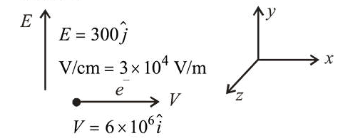# An electron is moving along +x direction with a velocityQuestion:

An electron is moving along $+x$ direction with a velocity of $6 \times 10^{6} \mathrm{~ms}^{-1}$. It enters a region of uniform electric field of $300 \mathrm{~V} / \mathrm{cm}$ pointing along $+y$ direction. The magnitude and direction of the magnetic field set up in this region such that the electron keeps moving along the $x$ direction will be :

1. (1) $3 \times 10^{-4} \mathrm{~T}$, along $+z$ direction

2. (2) $5 \times 10^{-3} \mathrm{~T}$, along $-z$ direction

3. (3) $5 \times 10^{-3} \mathrm{~T}$, along $+z$ direction

4. (4) $3 \times 10^{-4} \mathrm{~T}$, along $-z$ direction

Correct Option:

Solution:

(3) $\vec{E}=300 \hat{j} \mathrm{~V} / \mathrm{cm}=3 \times 10^{4} \mathrm{~V} / \mathrm{m}$

$\vec{V}=6 \times 10^{6} \hat{i}$$\vec{B}$ must be in $+z$ axis.

$q \vec{E}+q \vec{V} \times \vec{B}=0$

$E=V B$

$\therefore B=\frac{E}{V}=\frac{3 \times 10^{4}}{6 \times 10^{6}}=5 \times 10^{-3} \mathrm{~T}$

Hence, magnetic field $B=5 \times 10^{-3} \mathrm{~T}$ along $+z$ direction.